# Čerenkov radiation facts for kids

Kids Encyclopedia Facts

Cherenkov radiation, also known as Vavilov–Cherenkov radiation ( Russian: Черенков) is a type of electromagnetic radiation produced by charged particles when they pass through an optically transparent medium at a speed which is greater than the speed of light in that medium. (It doesn't violent the special relativity because, refractive index slows down the speed of light in a medium. So, the particle doesn't have to travel at the speed of light in a vacuum.) It is named after Pavel Alekseyevich Čerenkov who discovered this phenomenon in 1934 under the supervision of Sergey Vavilov. Igor Tamm and Ilya Frank developed a theory on this effect in 1937. Pavel Čerenkov, Igor Tamm and Ilya Frank shared the 1958 Nobel Prize in physics because of their contribution in cherenkov radiation.

## Theory

According to special relativity, a particle cannot move faster than the speed of light in a vacuum. However, when light travels in a transparent medium (such as water or glass), it moves more slowly than it would in a vacuum. This means that particles can actually move faster than the speed of light in certain mediums. When a particle with an electric charge moves faster than light in a medium which can be polarized, it causes the medium to send out photons (light particles) and thereby loses energy. The photons that are sent out can be measured, as they are simple light.

## Explanation

The formation of Cherenkov radiation is analogous to the bow wave caused by a power boat traveling faster than the speed of water waves or to the shock wave (sonic boom) produced by an airplane traveling faster than the speed of sound in air.

When an aircraft passes through the air, it creates a series of pressure waves in front of the aircraft and behind it. These waves travel at the speed of sound and, as the speed of the object increases, the waves get compressed as they cannot get out of each other's way quickly enough. When the aircraft gets the velocity of sound wave, the pressure waves get merge into a single shock wave. As the velocity keeps increasing, the single shock wave extends mostly to the rear and extends from the craft in a restricted widening cone. We hear this as sonic boom. Similarly, a bow wave forms when something moves through a fluid at a speed greater than the speed of a wave moving across the fluid. The mechanism of Cherenkov radiation is same but it occurs for light waves.

When a charged particle moves inside a polarizable medium, it excites some the ellectrons of that medium. As the excited ellectrons return to their ground state, they emits electromagnetic radiation. According to the Huygens principle, the emitted waves move out spherically at the phase velocity of that medium. If the particle moves faster than the speed of light in that medium, the emitted waves add up and at an angle with respect to the particle direction a radiation is emitted which is known as Cherenkov radiation.

Because nothing can move faster than light in a vacuum, there is no Cherenkov light in a vacuum. However, if we say that light in water moves only with 75% of its speed in vacuum, particles with very high energy are now able to move faster than light (through water) and create Cherenkov light. The reason Cherenkov light often appears blue is because its effect is proportional to the frequency, in that the higher the frequency, the higher the effect of the radiation. Because higher frequency light equates to shorter wavelengths, and blue light has one of the shortest wavelengths of visible light, Cherenkov light is usually blue.

### Emission angle with respect to the first image

In the first image, a charged particle (red) moves at a speed of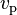$v_\text{p}$ where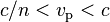$c/n < v_\text{p} < c$.

Ratio between the speed of the particle and the speed of light is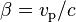$\beta=v_\text{p}/c$

In this medium, the velocity of light is (n is the refractive index)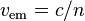$v_\text{em}=c/n$

Left side of this image is the initial point (t = 0) and the right side is the location of the particle after t time।

So, the distance passed by the particle in t time is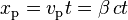$x_\text{p}=v_\text{p}t=\beta\,ct$

And the distance passed by the light is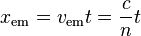$x_\text{em}=v_\text{em}t=\frac{c}{n}t$

So, the emission angle is (using trigonometry)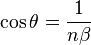$\cos\theta=\frac1{n\beta}$

We can also derive the emission angle from the second image too.

## Images for kidsČerenkov radiation Facts for Kids. Kiddle Encyclopedia.